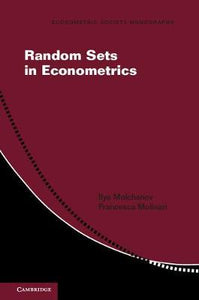# Random Sets in Econometrics by Molchanov, Ilya

• R 300.00
Unit price per
Tax included.

ISBN: 9781107548732

Cambridge University Press | 22 March 2019

Paperback | 194 pages

Description

Random set theory is a fascinating branch of mathematics that amalgamates techniques from topology, convex geometry, and probability theory. Social scientists routinely conduct empirical work with data and modelling assumptions that reveal a set to which the parameter of interest belongs, but not its exact value. Random set theory provides a coherent mathematical framework to conduct identification analysis and statistical inference in this setting and has become a fundamental tool in econometrics and finance. This is the first book dedicated to the use of the theory in econometrics, written to be accessible for readers without a background in pure mathematics. Molchanov and Molinari define the basics of the theory and illustrate the mathematical concepts by their application in the analysis of econometric models. The book includes sets of exercises to accompany each chapter as well as examples to help readers apply the theory effectively.